## 原理

• 模糊集

对于传统集合，一个元素要么属于这个集合，要么不属于这个集合，我们用1表示元素属于该集合，0表示元素不属于该集合。

模糊集合论认为一个集合可以不完全属于一个集合，用[0，1]之间的一个数来表示一个元素属于一个集合的程度，这个数叫做该集合的隶属度

• 模糊概念的清晰化

我们可以设定一个数（比如0.5）当一个元素的隶属度大于这个数时，我们就可以认为该元素时属于这个集合的

## 一级模糊综合评价

1. 确定因素集

对于员工的表现，需要从多个方面进行综合评判，如员工的业绩、工作态度、沟通能力、政治表现等。所有的这些因素构成了评价指标体系集合，即因素集，记为：
$$U={u_1,u_2,···,u_n }$$

2. 确定评语集

由于每个指标的评价值不同，往往会形成不同的等级。由各种不同决断构成的集合称为评语集，记为：
$$V={v_1,v_2,···v_n}$$

3. 确定各因素的权重

而通常不同的因素在综合评价中所起到的作用也是不同的，综合评价的结果很大程度上还依赖于各因素对综合评价所起的作用，就需要确定一个各因素之间的权重分配，它是U上的一个模糊向量，记为：
$$A=[a_1,a_2,···,a_n]$$
上式中，$a_i$为第$i$个因素的权重，且满足$\sum_{i=1}^na_i =1$

确定权重的方法有：Delphi法、加权平均法、众人评估法等

4. 确定模糊综合判断矩阵

对指标$u_i$来说，各个评语的隶属度为V上的模糊子集，对$u_i$的评判记为：
$$R_i = [r_{i1},r_{i2},···,r_{im}]$$
各指标的模糊判断矩阵为它是一个从U到V的模糊关系矩阵

5. 综合评判

如果有一个从U到V的模糊关系$R=(r_{ij})_{m\times n}$，那么利用R就可以得到一个模糊变换
$$T_R：F(U) \to F(v)$$
由此变换就可以得到模糊评价结果B = A · R

综合后的评判可以看作是V上的模糊向量，记为：
$$B=[b_1,b_2,···,b_m]$$

例子

某单位对员工的年终综合评定：

1. 因素集：U = {政治表现能力，工作能力，工作态度，工作业绩}

2. 评语集：V = {优秀，良好，一般，较差，差}

3. 确定各因素的权重：A = [0.25，0.2，0.25，0.3]

4. 确定模糊综合评判矩阵，最每个因素作出评价，通过群众评议打分确定：
$$R_1=[0.1,0.5,0.4,0,0]$$
说明：参与打分的群众中，由10%的人认为政治表现优秀，50%的人认为政治表现良好….同样的方法评判其他因素

得到评价矩阵：5. 模糊综合评价，进行矩阵合成运算：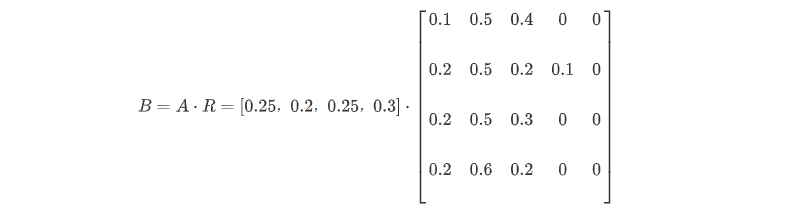得到：
$$B =[0.175,0.53,0.275.0.02,0]$$
取数值最大的评语作为综合评判结果为“良好”

## 多层次模糊综合评判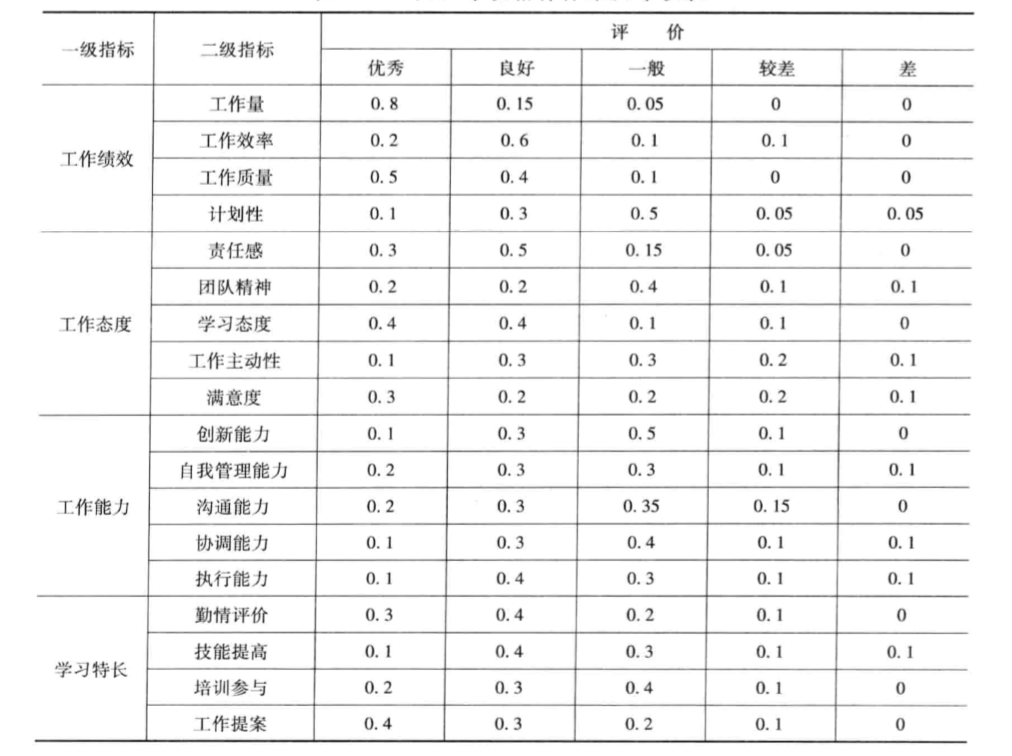1. 设专家设定指标权重，一级指标权重为：
$$A=[0.4,0.3,0.2,0.1]$$
二级指标权重为：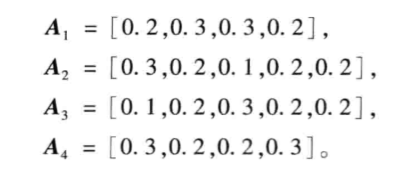1. 对各个因素进行一级模糊综合评判得到：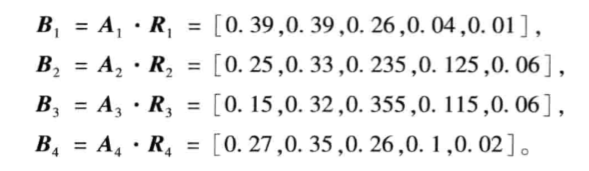2. 得到二级评判为：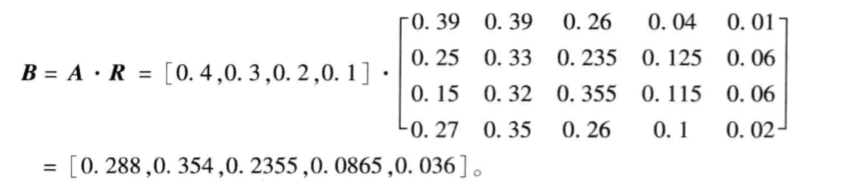data1.txt